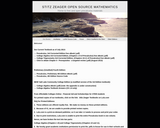Updating search results...

# 3 Results

View
Selected filters:
• Stitz-Zeager Open Source MathematicsConditional Remix & Share Permitted
CC BY-NC-SA
Rating
0.0 stars

College Algebra is an introductory text for a college algebra survey course. The material is presented at a level intended to prepare students for Calculus while also giving them relevant mathematical skills that can be used in other classes. The authors describe their approach as "Functions First," believing introducing functions first will help students understand new concepts more completely. Each section includes homework exercises, and the answers to most computational questions are included in the text (discussion questions are open-ended).

Subject:
Algebra
Mathematics
Material Type:
Textbook
Provider:
Stitz-Zeager Open Source Mathematics
Author:
Carl Stitz
Jeff Zeager
07/15/2011Conditional Remix & Share Permitted
CC BY-NC-SA
Rating
0.0 stars

This book was designed as an introductory trigonometry textbook for college students with the explicit goal of reducing textbook costs.

Subject:
Algebra
Mathematics
Trigonometry
Material Type:
Textbook
Provider:
Stitz-Zeager Open Source Mathematics
Author:
Carl Stitz
Jeff Zeager
07/15/2011Conditional Remix & Share Permitted
CC BY-NC
Rating
0.0 stars

A casual glance through the Table of Contents of most of the major publishers' College Algebra books reveals nearly isomorphic content in both order and depth. Our Table of Contents shows a different approach, one that might be labeled “Functions First.” To truly use The Rule of Four, that is, in order to discuss each new concept algebraically, graphically, numerically and verbally, it seems completely obvious to us that one would need to introduce functions first. (Take a moment and compare our ordering to the classic “equations first, then the Cartesian Plane and THEN functions” approach seen in most of the major players.) We then introduce a class of functions and discuss the equations, inequalities (with a heavy emphasis on sign diagrams) and applications which involve functions in that class.

The material is presented at a level that definitely prepares a student for Calculus while giving them relevant Mathematics which can be used in other classes as well. Graphing calculators are used sparingly and only as a tool to enhance the Mathematics, not to replace it. The answers to nearly all of the computational homework exercises are given in thetext and we have gone to great lengths to write some very thought provoking discussion questions whose answers are not given. One will notice that our exercise sets are much shorter than the traditional sets of nearly 100 “drill and kill” questions which build skill devoid of understanding. Our experience has been that students can do about 15-20 homework exercises a night so we very carefully chose smaller sets of questions which cover all of the necessary skills and get the students thinking more deeply about the Mathematics involved.

Subject:
Calculus
Mathematics
Material Type:
Textbook
Provider:
Stitz-Zeager Open Source Mathematics
Author:
Carl Stitz
Jeff Zeager